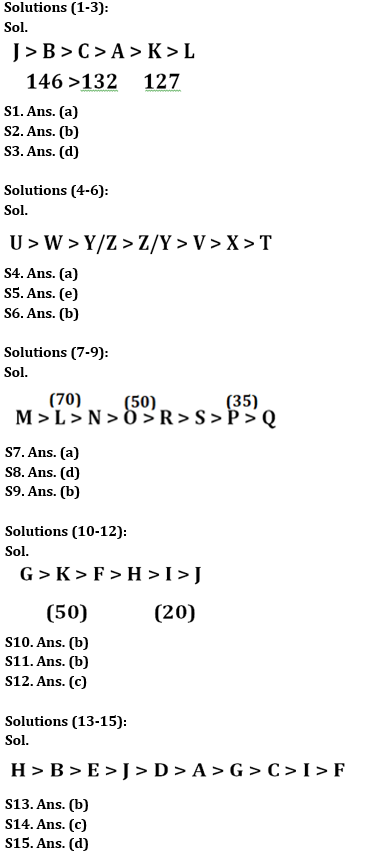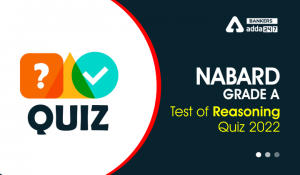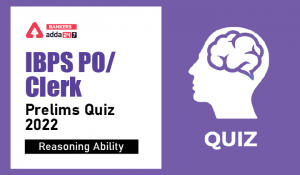Latest Banking jobs   »

# Reasoning Ability Quiz For IBPS Clerk Prelims 2022- 11th July

Directions (1-3): Answer the following question based on the information given below:
Six people J, K, L, A, B, and C are of different heights. The difference between B’s height and C’s height is 14cm. J is taller than B. B is taller than C who is not the shortest. Two people are shorter than A. K is shorter than C but not the shortest. The height of the second tallest person is 146cm. The height of C is a multiple of 4. The height of the second shortest person is 127cm.

Q1. Who is the 2nd tallest?
(a) B
(b) J
(c) K
(d) L
(e) None of these

Q2. How many people are shorter than K?
(a) Two
(b) One
(c) Four
(d) Three
(e) None of these

Q3. What could be the height of A?
(a) 122cm
(b) 138cm
(c) 141cm
(d) 130cm
(e) None of these

Directions (4-6): Answer the following question based on the information given below:
Seven students T, U, V, W, X, Y & Z scored different marks in an examination. V scored more than only T & X. W scored less than only U. X does not score the least. The one who scored the third highest marks scored 90 marks & X scored 60 marks. The student who scored the maximum marks among them scored 20 marks more than Y’s marks.

Q4. Who among the following scored the least marks?
(a) T
(b) Z
(c) Y
(d) W
(e) None of the above

Q5. How many persons scored more marks than Y?
(a) Two
(b) Three
(c) Four
(d) Either ‘three’ or ‘four’
(e) Either ‘two’ or ‘three’

Q6. What may be the possible marks scored by W if Y & Z scored 90 & 80 respectively?
(a) 81
(b) 91
(c) 88
(d) 89
(e) None of the above

Directions (7-9): Answer the following question based on the information given below:
Eight persons L, M, N, O, P, Q, R & S are of different age. Only Q is younger than P whose age is 35 years. Only M is older than L whose age is 70 years. O is younger than N but older than R. S is younger than R. O’s age is 50 years.

Q7. Who among the following is 2nd eldest among all?
(a) L
(b) N
(c) M
(d) O
(e) None of the above

Q8. How many persons are younger than L?
(a) Three
(b) Four
(c) Five
(d) Six
(e) None of the above

Q9. What may be the possible age of N?
(a) 72years
(b) 65years
(c) 71 years
(d) 48 years
(e) None of these

Directions (10-12): Answer the following question based on the information given below:
There are six items F, G, H, I, J & K. Each item has different weight. F is heavier than I but lighter than K. The weight of second heaviest item is 50kg. The weight of second lightest item is 20kg. The weight of G is more than the weight of I and J which is lightest. K is not the heaviest item. H is lighter than F but heavier than I.

Q10. How many persons are heavier than F?
(a) One
(b) Two
(c) Three
(d) Four
(e) None of the above

Q11. If the difference between the weights of F and I is 25kg, then what will be the possible weight of H?
(a) 15
(b) 25
(c) 55
(d) 65
(e) None of the above

Q12. Who among the following person is 3rd heaviest?
(a) K
(b) G
(c) F
(d) H
(e) None of the above

Directions (13-15): Answer the following question based on the information given below:
Ten persons A, B, C, D, E, F, G, H, I, and J have different heights. C is taller than two persons. B is shorter than only H. J is taller than D but shorter than E. A is taller than G who is taller than I and F. D is not shorter than A. I is not the shortest person.

Q13. How many persons are shorter than J?
(a) Five
(b) Six
(c) Three
(d) Four
(e) None of the above

Q14. Who among the following is the 4th shortest person?
(a) A
(b) I
(c) G
(d) F
(e) None of the above

Q15. How many persons are taller than D?
(a) Five
(b) Six
(c) Three
(d) Four
(e) None of the above

Solutions#### Congratulations!Incorrect details? Fill the form again here

•Reasoning Ability Quiz For IBPS RRB PO C...
•Reasoning Ability Quiz For NABARD Grade ...
•Reasoning Ability Quiz For IBPS Clerk/PO...
•Reasoning Ability Quiz For IBPS RRB PO C...
•Reasoning Ability Quiz For IBPS Clerk Pr...
•Reasoning Ability Quiz For IBPS RRB PO C...